Question

# For each run of the iodine clock reaction, 5.00 mL of a 2.02×10-2 M arsenious acid...

For each run of the iodine clock reaction, 5.00 mL of a 2.02×10-2 M arsenious acid solution is added to the reaction mixture. The total volume of the reaction mixture is 100.0 mL. What is the initial rate of the reaction if the solution turns blue after 68 seconds?

Given information:

• Initial rate of arsenious acid is 2.02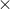10-2 M
• Time is 68 seconds

The iodine clock reaction is shown below: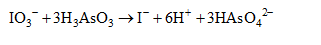The initial rate for arsenious acid at 68 seconds is calculated as follows: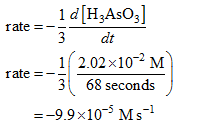Negative sign only shows the decomposition of arsenious acid. Therefore, the initial rate for arsenious acid at 68 seconds is 9.9 × 10-5 M s-1.

#### Earn Coins

Coins can be redeemed for fabulous gifts.

Similar Homework Help Questions
• ### equations that may be useful are: 2I(-)+SO2O8(2-)->I2+2SO4(2-) and I2+2S2O3(2-)->2I(-)+S4O6(2-) THE IODINE CLOCK- REACTION KINETICS IN-LAB GUIDELINES...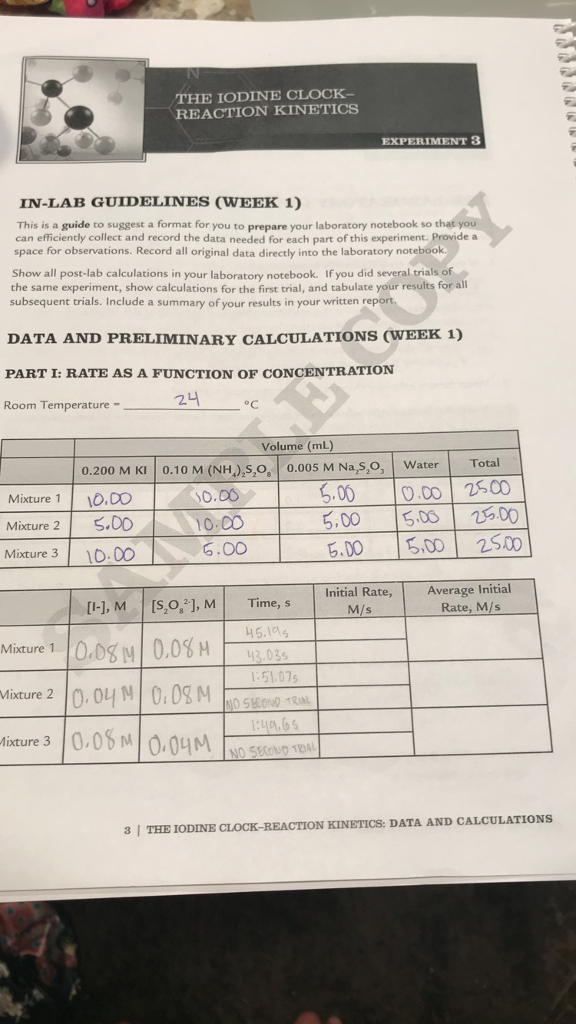equations that may be useful are: 2I(-)+SO2O8(2-)->I2+2SO4(2-) and I2+2S2O3(2-)->2I(-)+S4O6(2-) THE IODINE CLOCK- REACTION KINETICS IN-LAB GUIDELINES (WEEK 1) This is a guide to suggest a format for you to prepare your laboratory notebook so that you can efficiently collect and record the data needed for each part of this experiment. Provide a space for observations. Record all original data directly into the laboratory notebook. Show all post-lab calculations in your laboratory notebook. If you did several trials of the same...

• ### A solution was prepared by adding 12.00 ml 3.00 mL of 0.130 M KNO_3, 5.00 mL...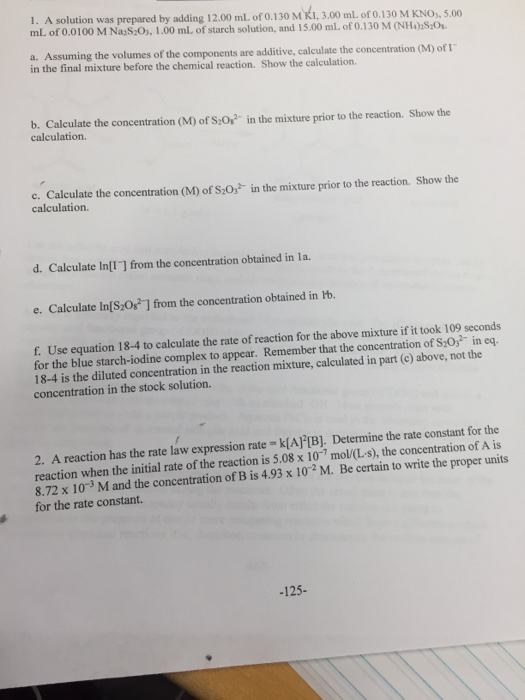A solution was prepared by adding 12.00 ml 3.00 mL of 0.130 M KNO_3, 5.00 mL of 0.0100 M Na_2S_2O_3, 1.00 mL of starch solution, and 15.00 mL of 0.130 M (NH_4)_2 S_2O_8. Assuming the volumes of the components are additive, calculate the concentration (M) of I^- in the final mixture before the chemical reaction. Show the calculation. Calculate the concentration (M) of S_2O_8^2- in the mixture prior to the reaction. Show the calculation. Calculate the concentration (M) of S_2O_3^2-...

• ### How do I calculate the isolated moles and molarity of the 0.2M KIO3?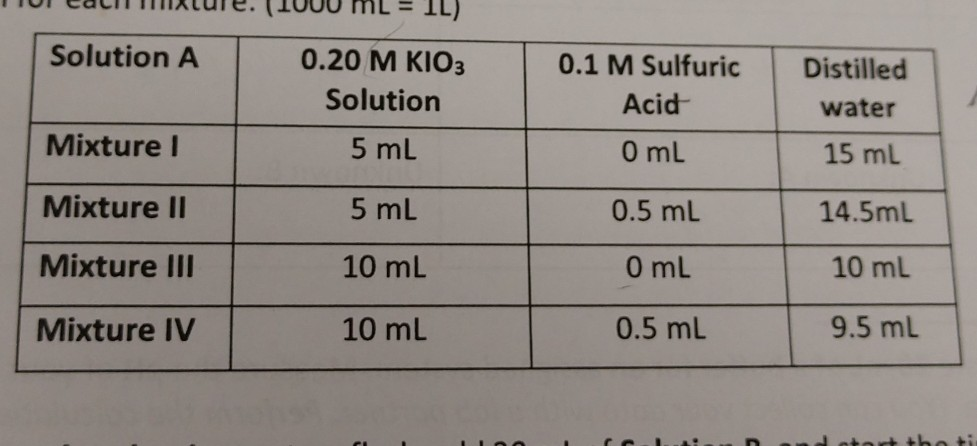how do I calculate the isolated moles and molarity of the 0.2M KIO3? 0.1 M Sulfuric Distilled Solution A Mixturel Mixture II Mixture III Mixture IV 0.20 M KIO3 Solution 5 mL 5 mL 10 mL 10 mL Acid 0 mL 0.5 mL 0 mL 0.5 mL water 15 ml 14.5mL 10 mL 9.5 mL Lalculations: I. (6pt) Calculate the total number of moles of iodate ion present in each 0.2 M KlO3 solution. Calculate the molarity of iodate ion...

• ### Lab 20A lodination of Acetone Concentration SJBoylan2018 RATIO METHOD TO DETERMINE REACTION RATE MODEL You add...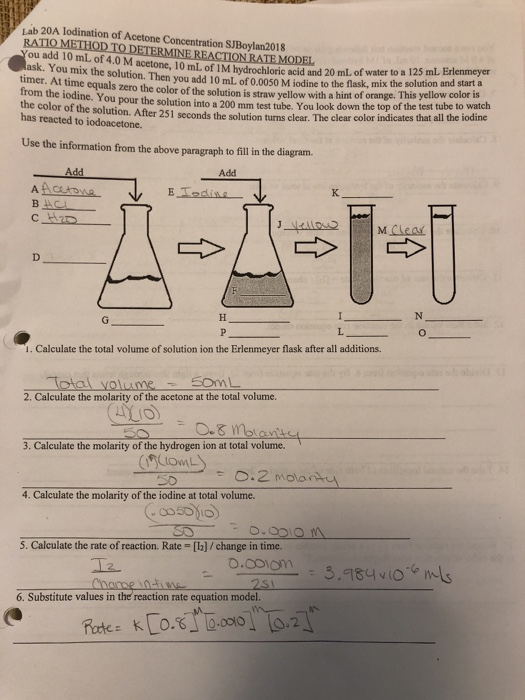Lab 20A lodination of Acetone Concentration SJBoylan2018 RATIO METHOD TO DETERMINE REACTION RATE MODEL You add 10 mL of 4.0 M acetone, 10 mL of 1M hydrochloric acid and 20 mL of water to a 125 mL Erlenmeyer ask. You mix the solution. Then you add 10 mL of 0.0050 M iodine to the flask, mix the solution and start a timer. At time equals zero the color of the solution is straw yellow with a hint of orange. This...

• ### In iodine clock experiment prelab 2.During the experiment, after each trial, where will the reaction mixture...

In iodine clock experiment prelab 2.During the experiment, after each trial, where will the reaction mixture and runoff of rinsing your glassware be collected ? 3. If different than your answer to question 2, after the experiment where will the reaction mixture and runoff of rinsing your glassware be collected?

• ### A student studied the clock reaction described in this experiment. She set up Reaction Mixture 2...

A student studied the clock reaction described in this experiment. She set up Reaction Mixture 2 by mixing 20 mL 0.010 M KI, 10 mL .001 M Na2S2O3, 10 mL .040 M KBrO3, and 10 mL 0.10 M HCl using the procedure given. It took 45 seconds for the color to turn to blue. a. She found the concentration of each reactant in the reacting mixture by realizing that the number of moles of each reactant did not change when...

• ### Table 2. Conditions for Reaction Rate Study. Volume solution, mL Vessel Solution Mixture 1 Mixture 2...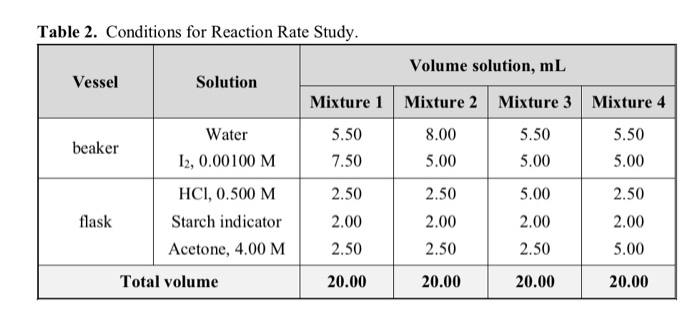Table 2. Conditions for Reaction Rate Study. Volume solution, mL Vessel Solution Mixture 1 Mixture 2 Mixture 3 Mixture 4 beaker 5.50 7.50 8.00 5.00 5.50 5.00 5.50 5.00 Water 12, 0.00100 M HCI, 0.500 M Starch indicator Acetone, 4.00 M 2.50 2.50 2.50 2.00 flask 2.00 2.50 5.00 2.00 2.50 2.00 2.50 5.00 Total volume 20.00 20.00 20.00 20.00 Table 2. Reaction Conditions and Rate of Reaction. Initial Mixture Initial [H], Initial , [CH3COCH3] Number M M M -A,...

• ### A student mixes 5.00 mL of 2.00 times 10^-3 M Fe(NO_3)_3 with 5.00 mL of 2.00...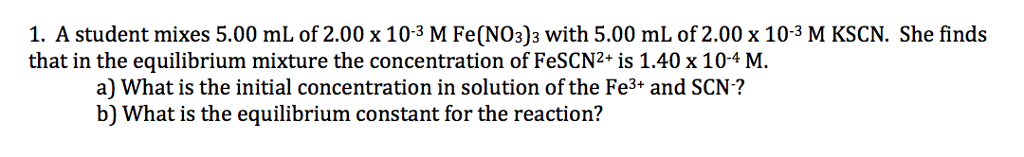A student mixes 5.00 mL of 2.00 times 10^-3 M Fe(NO_3)_3 with 5.00 mL of 2.00 times 10^-3 M KSCN. She finds that in the equilibrium mixture the concentration of FeSCN2^+ is 1.40 times 10^-4 M. a) What is the initial concentration in solution of the Fe^3+ and SCN^-? b) What is the equilibrium constant for the reaction?

• ### Question below: A solution containing 5 mL of each 4 M acetone, 1 M HCl, and...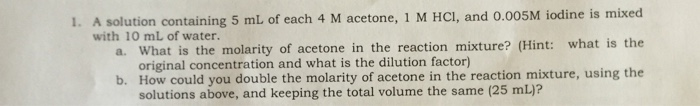Question below: A solution containing 5 mL of each 4 M acetone, 1 M HCl, and 0.005M iodine is mixed with 10 mL of water. What is the molarity of acetone in the reaction mixture? How could you double the molarity of acetone in the reaction mixture, using the solutions above, and keeping the total volume the same (25 mL)?

• ### An iodine-clock kinetics experiment was performed by varying the composition of reactant solutions and measuring the...

An iodine-clock kinetics experiment was performed by varying the composition of reactant solutions and measuring the resulting rates of reaction. Initial Rate Experiment 1 Initial Rate Experiment 2 Initial Rate Experiment 3 Test tube contents 10.0 mL 0.10 M NaI(aq) 10.0 mL 0.10 M Na2S2O8(aq) 5.0 mL 0.0050 M Na2S2O3(aq) 1.0 mL starch solution 10.0 mL 0.10 M NaI(aq) 5.0 mL 0.10 M Na2S2O8(aq) 5.0 mL 0.10 M Na2SO4(aq) 5.0 mL 0.0050 M Na2S2O3(aq) 1.0 mL starch solution 5.0 mL...